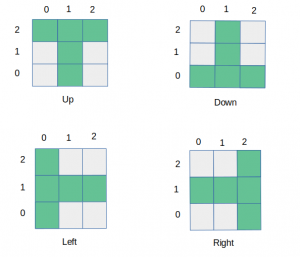# Check if the given 2-D points form T-shape or not

• Difficulty Level : Medium
• Last Updated : 21 Jan, 2021

Given the coordinates of 5 2-dimensional points, check if they form a closed T shape. Print ‘Yes’ if they form T shape and ‘No’ otherwise. Note: Coordinates should be distinct and integers.
There are 4-types of T shaped formations possible according to the given conditions:Examples:

```Input: [[7, 5], [8, 5], [6, 5], [7, 7], [7, 6]]
Output: Yes

Input: [[0, 0], [1, 0], [2, 0], [1, -1], [1, -2]]
Output: Yes```

Approach:

1. Consider the first point in the given list as the center (x, y) (i.e intersection of the two lines that form a T-shape).
2. Then check if all the points which are needed to form a T-shape of which (x, y) are the center are present in the list of given points or not.
3. Check this for all 4 possible patterns of T-shape.

Below is the implementation of the above approach:

## Python3

 `# Python3 code to check if given 5` `# 2-D points form T-shape or not`   `# Import the function to initialize the` `# dictionary with a specific value` `from` `collections ``import` `defaultdict`   `# This function checks if the points` `# form T-shape pointing up`     `def` `isUpDirected(point, x, y):`   `    ``return` `(point[(x``-``1``, y)] ``and` `            ``point[(x, y)] ``and` `            ``point[(x ``+` `1``, y)] ``and` `            ``point[(x, y``-``1``)] ``and` `            ``point[(x, y``-``2``)])`   `# This function checks if the points` `# form T-shape pointing down`     `def` `isDownDirected(point, x, y):`   `    ``return` `(point[(x``-``1``, y)] ``and` `            ``point[(x, y)] ``and` `            ``point[(x ``+` `1``, y)] ``and` `            ``point[(x, y ``+` `1``)] ``and` `            ``point[(x, y ``+` `2``)])`   `# This function checks if the points` `# form T-shape pointing left`     `def` `isLeftDirected(point, x, y):`   `    ``return` `(point[(x, y ``+` `1``)] ``and` `            ``point[(x, y)] ``and` `            ``point[(x, y``-``1``)] ``and` `            ``point[(x ``+` `1``, y)] ``and` `            ``point[(x ``+` `2``, y)])`   `# This function checks if the points` `# form T-shape pointing right`     `def` `isRightDirected(point, x, y):`   `    ``return` `(point[(x, y ``+` `1``)] ``and` `            ``point[(x, y)] ``and` `            ``point[(x, y``-``1``)] ``and` `            ``point[(x``-``1``, y)] ``and` `            ``point[(x``-``2``, y)])`     `# This function checks if given points` `# form a T-shape or not` `def` `solve(grid):`   `    ``# Initialize the dictionary with False value` `    ``point ``=` `defaultdict(``lambda``: ``False``)` `    ``flag ``=` `False`   `    ``for` `i ``in` `range``(``len``(grid)):`   `        ``# Assign True value to the points which` `        ``# are present in the given list` `        ``point[(grid[i][``0``], grid[i][``1``])] ``=` `True`   `    ``for` `i ``in` `range``(``len``(grid)):`   `        ``# Check if the given points form any of the` `        ``# 4 possible T-shaped formations` `        ``if` `isUpDirected(point, grid[i][``0``], grid[i][``1``]) ``or` `           ``isDownDirected(point, grid[i][``0``], grid[i][``1``]) ``or` `            ``isLeftDirected(point, grid[i][``0``], grid[i][``1``]) ``or` `            ``isRightDirected(point, grid[i][``0``], grid[i][``1``]):`   `            ``flag ``=` `True` `            ``break`   `    ``if` `flag ``=``=` `True``:` `        ``return` `'Yes'` `    ``else``:` `        ``return` `'No'`     `print``(solve([[``7``, ``5``], [``8``, ``5``], [``6``, ``5``], [``7``, ``7``], [``7``, ``6``]]))` `print``(solve([[``0``, ``0``], [``1``, ``0``], [``2``, ``0``], [``1``, ``-``1``], [``1``, ``-``2``]]))`

Output:

```Yes
Yes```

Time Complexity: O(1)

My Personal Notes arrow_drop_up
Recommended Articles
Page :